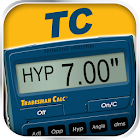All Android applications categories

All Android games categories22 9.4

9.4 Users
rating

## Screenshots

Description

Trades Math and Conversion Calculator by Calculated Industries

All the functionality and solutions (see list below) of the handheld Tradesman Calc calculator optimized for the Android user. And while perhaps obvious, if you change from an Android device to an iPhone or iPad, you WILL need to buy a new license as they are incompatible.

The Tradesman Calc for Android devices is specifically designed to be useful in trades requiring technical math, including Welding, Metal Fabrication, Engineering, Automotive Service Technology, Aviation and Drafting - virtually any skilled trade requiring applied math skills.

This powerful, easy-to-use app helps tradesmen on the job whenever they need to use or calculate dimensional math, fractions (ruler, proper, improper, and mixed), unit conversions, ratios and proportions, trigonometry/trig and plane geometry (right triangles/angles, circular and polygonal shapes). In addition, the Tradesman Calc app has a wide range of powerful math functions, including the ability to enter math equations using order of operations. And you can work with exponents, square roots, reciprocals and more.

The app also has a wide range of powerful math functions, including the ability to enter math equations using order of operations. And you can work with exponents, square roots, reciprocals and more.

The Tradesman Calc app is easy-to-teach and easy-to-learn, making it ideal for instructors helping students in career and technical education programs develop and expand their applied and practical math skills.

FEATURES
Created by Calculated Industries, the originator of the award-winning Construction Master Calculators
Built-in help – just press and hold key
All the functionality of the Tradesman Calc handheld calculator and optimized for the Android device user
Entry editing backspace key
Complete User’s Guide available free at www.calculated.com

DEDICATED FUNCTIONS
Work in Architectural (ruler) fractions (1/4 inch, 1/16 inch, etc.), proper fractions (2/3), mixed number fractions (1 2/3) and improper fractions (5/3)
Solve dimensional math problems, and convert between U.S. and metric dimensional math units for easy conversions.
Right Triangle/Angle Math: Enter and calculate Angle, Hypotenuse, Opposite and Adjacent side lengths and make solving right triangles easy
Trigonometric/Trigonometry/Trig Functions: Solve angle and side trigonometry problems using Sine, Cosine, Tangent, Arcsine, Arccosine, Arctangent
Circle/Arc: Enter and calculate Area, Circumference, Radius, Diameter, Arc Angle, Arc Length, Chord Length, Arc Height
Ratios and Proportions: Calculate x, y values and calculate proportional values easily with the ratio and proportion functionality
Hexagon/Polygon: Calculate Exterior Angle, Interior Angle, Side Length, Outside Diameter, Inside Diameter, Perimeter and Area
Enter math equations using order of operations
Work with exponents

Great for: Students, Instructors and those active in the Trades – Welding, Metal Fabrication, Engineering, Automotive Service Technology, Aviation and Drafting

from 22 reviews

"Awesome"

9.4